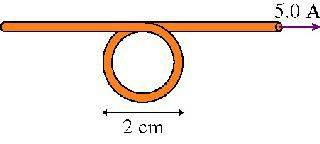# Magnetic field at center of loop

1. What is the magnetic field at the center of the loop in the figure ?2. Ampere's Law

3. If the surface integral of B*ds = Bl = 2*pi*d = u0*I:

B = u0I / (2pi*d) = 5*5-5 T, which is wrong.

rl.bhat
Homework Helper
B = u0I / (2pi*d)
This formula is for a long straight conductor.
In the given problem in addition to the straight conductor, one circular loop is also present.
Find the field at the center of the circular coil and add it to the above.

Still not getting it.

I calculated B_loop = u0IR2/[2(z2+R2)3/2]

With z = 0 because we're at the center of the loop, it reduces to B = u0I/2R = 3.15*10-4.

I know the answer is 4.14*10-4 so I muse be making some sort of small calculation error...

Could somebody also explain, conceptually, why I would calculate B for a straight conductor and a loop and add them? For example, why would I calculate B for the full length of the conductor if some of it isn't straight (i.e. is looped)? Or is that part of my mistake here?

rl.bhat
Homework Helper

B = u0I / (2pi*d) = 5*5-5 T

Check this calculation. Here d = 1 cm.

why is d = 1cm and not 2cm? Is it the radius of the loop? Why not call it r?

ideasrule
Homework Helper
d is the distance from the straight wire, which is 1 cm.

ideasrule
Homework Helper
Could somebody also explain, conceptually, why I would calculate B for a straight conductor and a loop and add them? For example, why would I calculate B for the full length of the conductor if some of it isn't straight (i.e. is looped)? Or is that part of my mistake here?

Suppose that you could see the electrons flowing. The straight part of the wire in this problem looks exactly like a normal straight wire: the electrons are flowing in a straight line from one end to the other. The circular part looks exactly like a normal circular current loop: the electrons seem to be going in circles. Due to the principle of superposition, you can calculate the magnetic field induced by the straight part and the circular part and add them to get the resultant field.

Thanks